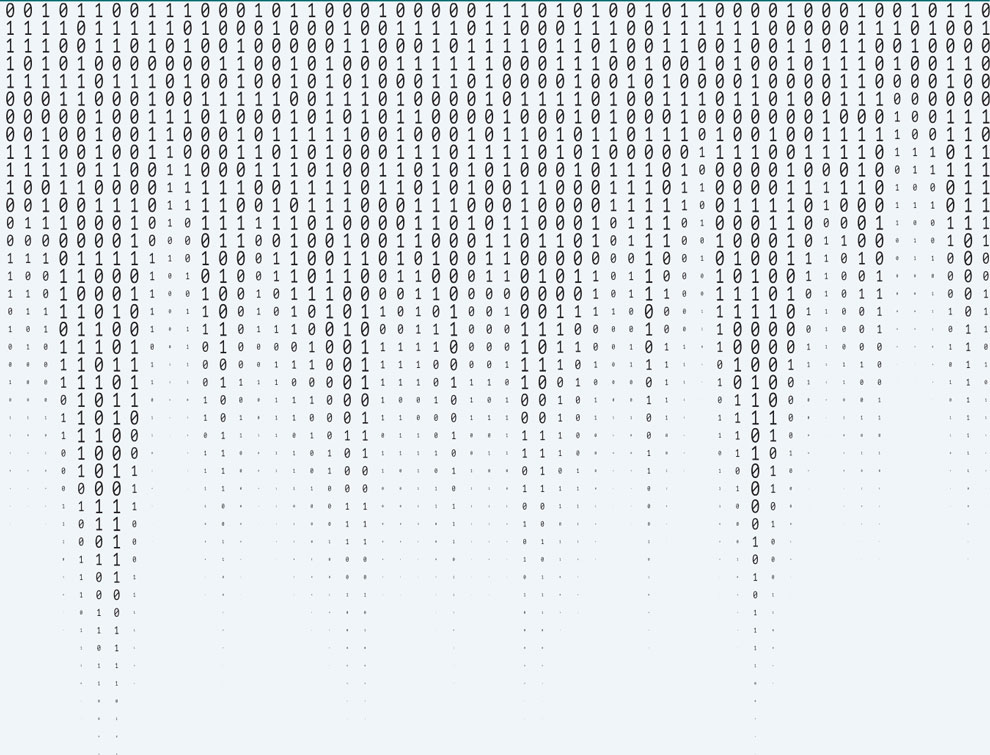# Identifiable SequencesStephen Mildenhall suggested the following puzzle. Create two sequences of strictly increasing positive integers, A(n) and B(n) with n = 1,2,…. These sequences must be such that any positive integer M is the sum M = A(i) + B(j) for at most one pair i,j. Note, however, not all M need to be such a sum. When A and B together have this property, they are called identifiable.

Here is a magic trick to illustrate how identifiable sequences might be used. You will give list A to Person A and list B to Person B and ask them each to randomly select one number. They confer and tell you only the sum. With identifiable sequences you can magically tell them which number each of them chose.

One pair of identifiable sequences is A(1) = 1, B(n) = 2 A(n), A(n + 1) = 2 B(n). Some example unique sums from these sequences are: 3 = 1 + 2. 6 = 4 + 2. 9 = 1 + 8, 12 = 4 + 8, … But A and B grow very rapidly with n.

In contrast, the slow growing sequences A(n) = 2n and B(n) = 2n + 1 are not identifiable. For example, 7 = 2 + 5 = 4+ 3.

In general, we expect only a small proportion of all the positive integers can be formed as A(i) + B(i) when A and B are identifiable.

The challenge is to construct the slowest growing pair of identifiable sequences. Assume A(1) = 1 and B(1) = 2. What do you propose for a pair A(n) and B(n)? How do you compute them as efficiently as possible? Can you say anything about their growth rates as functions of n? Polynomial? Exponential? What is A(100)? A(200)? Extra credit: A(1000)? You may need a computer.